Reinforcement Learning: An Introduction 学习笔记（2）

[TOC]

Chapter 4 Dynamic Programming

Policy Evaluation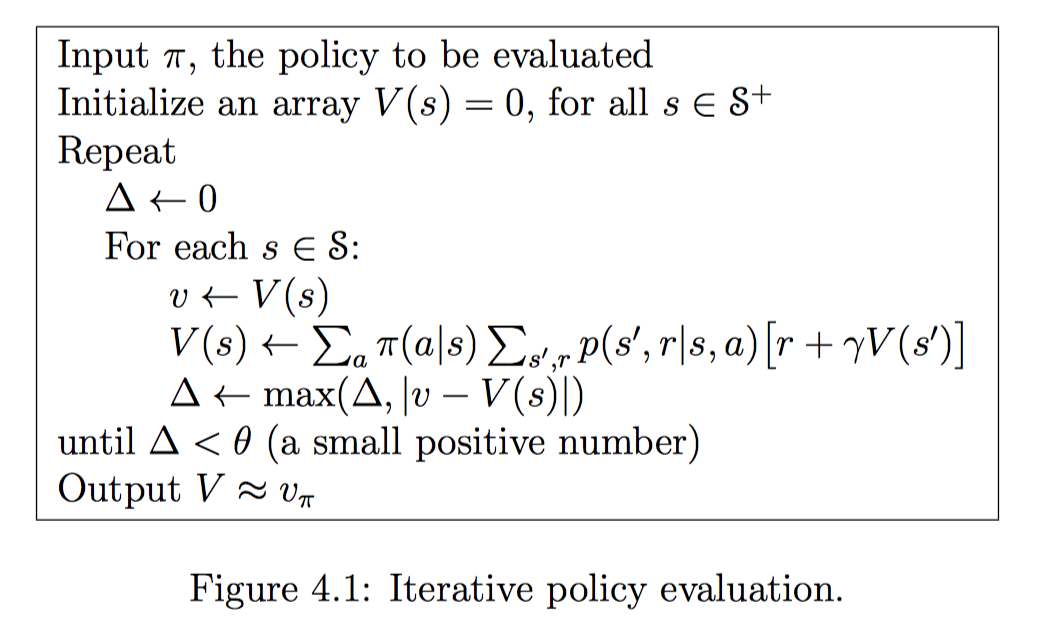Policy Improvement

$$v_{\pi’}(s) \ge v_{\pi}(s)$$

\begin{equation} \begin{aligned} \pi’(s) &= \mathop{\arg\max}{a}q\pi(s,a) \ &= \mathop{\arg\max}a\mathbb{E}\big[R{t+1} + \gamma v_\pi(S_{t+1})\ | \ S_t=s, A_t=a \big] \end{aligned} \end{equation}

Policy Iteration

$$\pi_0 \overset{\text{E}}{\rightarrow} v_{\pi_0} \overset{\text{I}}{\rightarrow} \pi_1 \overset{\text{E}}{\rightarrow} v_{\pi_1} \overset{\text{I}}{\rightarrow} \pi_2 \overset{\text{E}}{\rightarrow} \dots \overset{\text{I}}{\rightarrow} \pi_ \overset{\text{E}}{\rightarrow} v_{}$$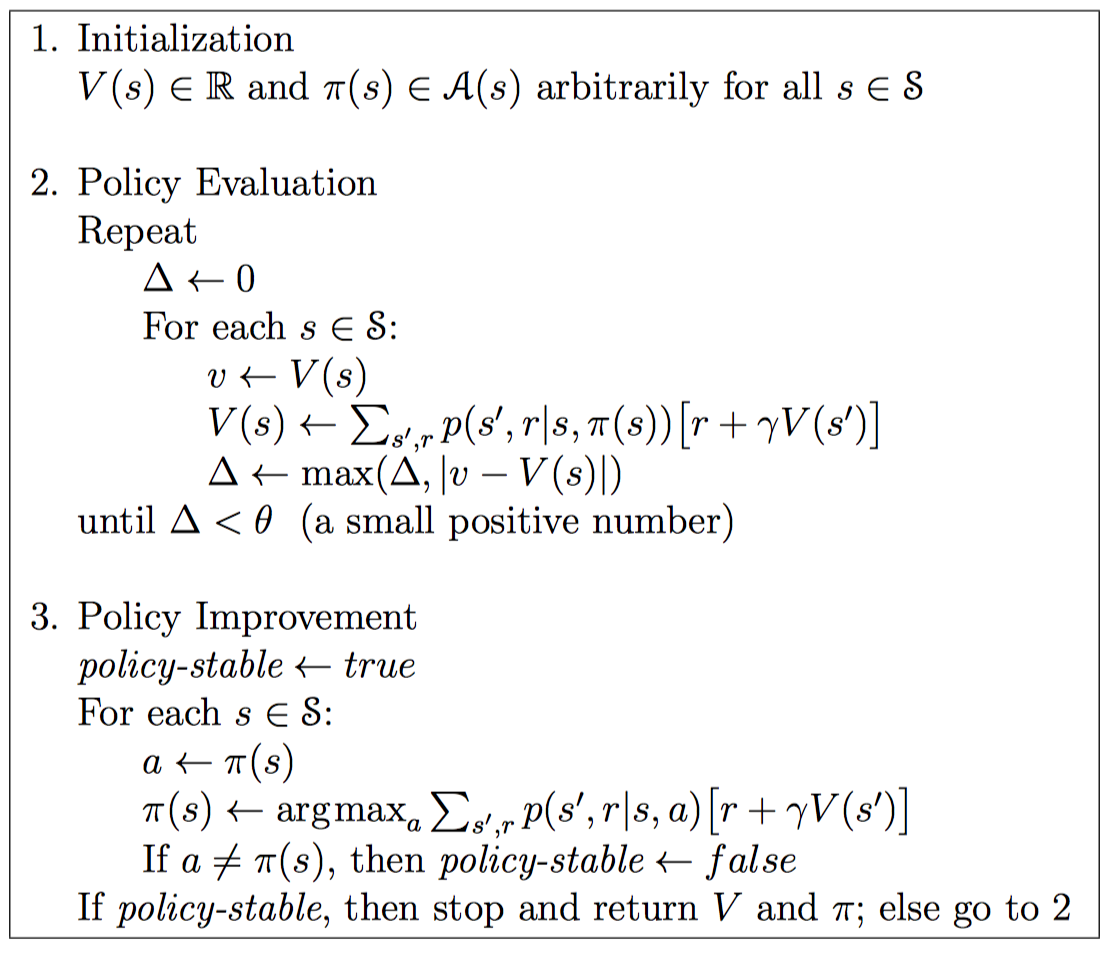Value Iteration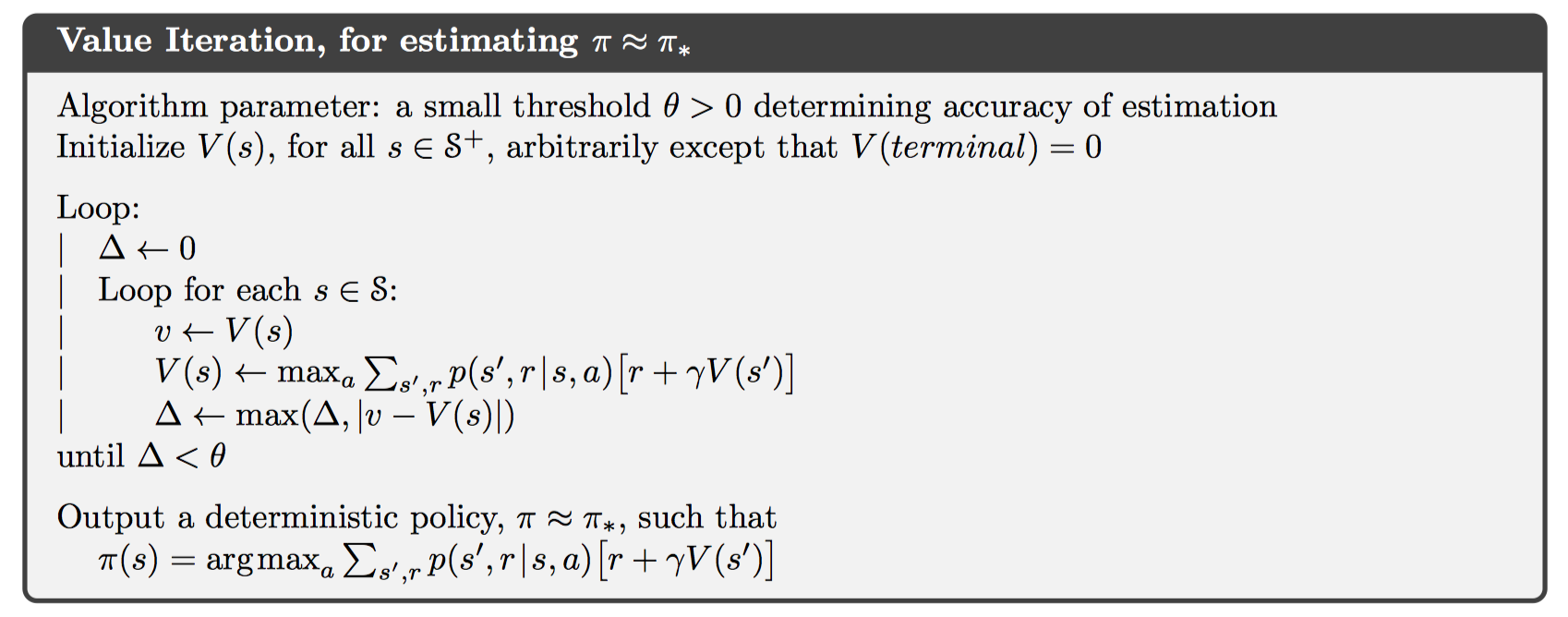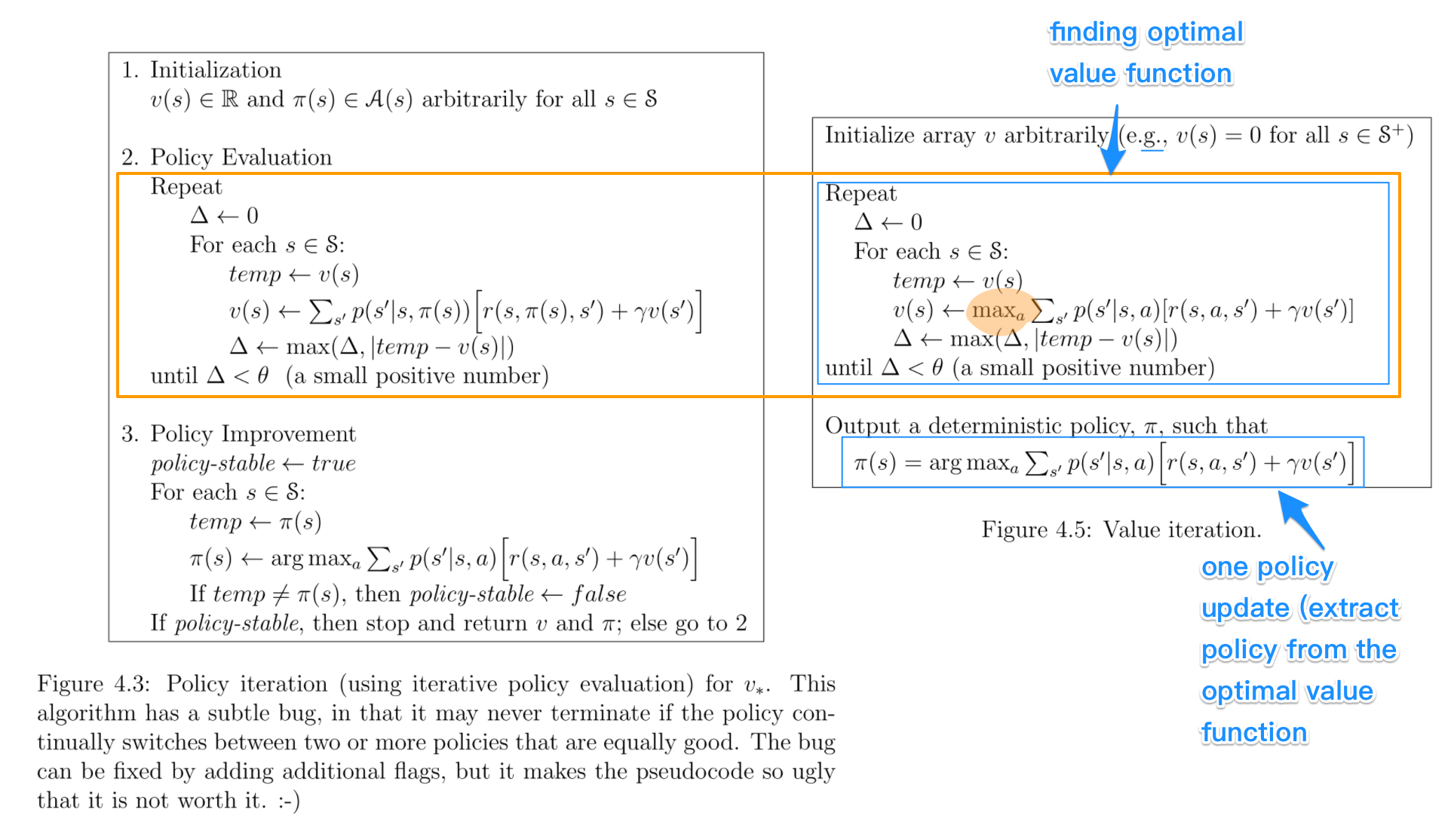Chapter 5 Monte Carlo Methods

MC被广泛地应用于含有随机的情景下。MC与多臂赌博机问题有很多相似之处 – 都是通过采样、取均值的方法对state-action二元组进行估计；主要的不同是这里存在着多种状态，每一个状态都单独是一个多臂赌博机问题，并且各个状态之间是相互关联的。也就是说，在同一阶段，当前状态采取的行为将会影响下一个状态采取的行为获得的收益。但是相对于DP方法，各个状态之间又是相互独立的，原因是在DP中估计值函数需要一个状态之后的所有状态，而蒙特卡洛方法只需要一个状态而已，也就是采样到的那一个收益。

• 能够直接从经验中学习，而不需要建立理论模型
• 能够避免$p(s’, r|s, a)$的计算
• 能够只关注重要的状态而不去关心其它次要状态

Monte Carlo Prediction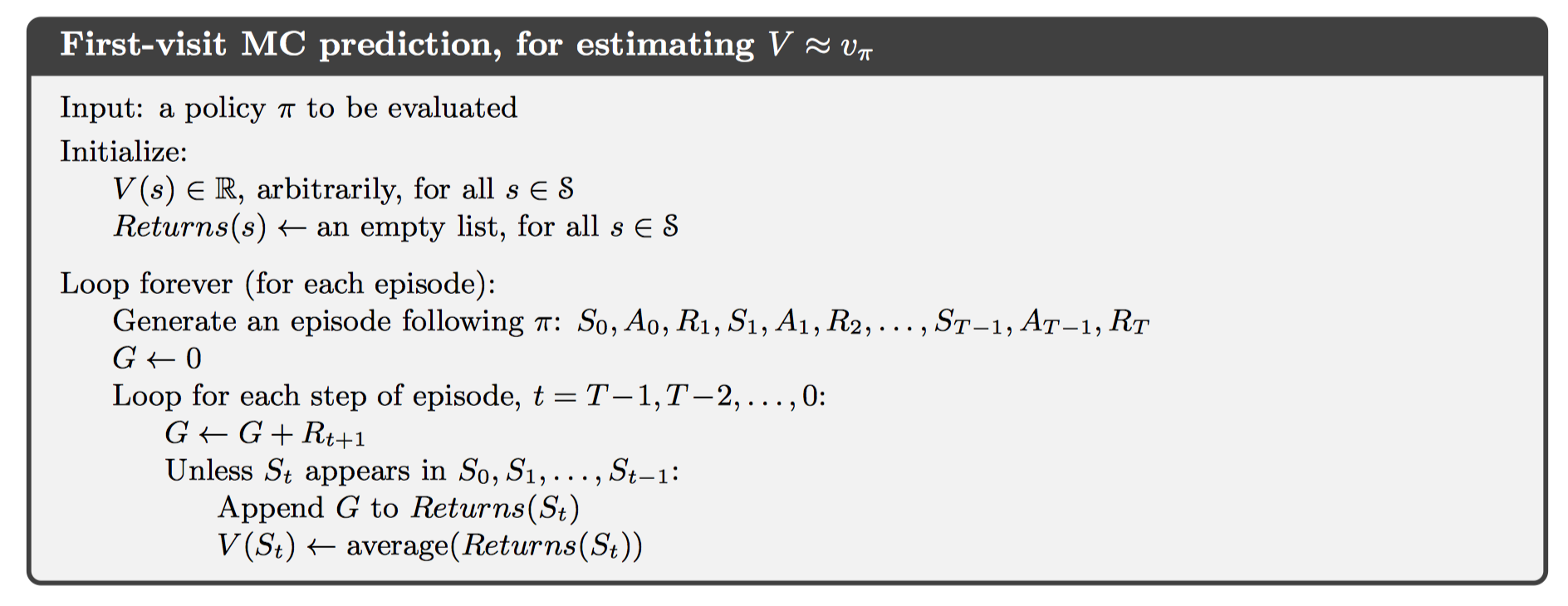• 对于收益的计算是逆序进行的，其实是一种类似前缀和的思想。我们关心的所谓从一个状态出发的收益，正是pre[n] - pre[i]，这就是逆序求和的原因所在。
• unless在这里判断的，是要看该状态在本阶段是否已经计算过。因此本算法是first-visit MC method

MC Estimating for Action Values

• 在确定性策略的情况下，有些状态行为也许永远也访问不到。
• 我们使用的数据不可能包含有无限组数据，因此最终的结果一定是渐进收敛到真值，但不是真值。

MC Exploring Start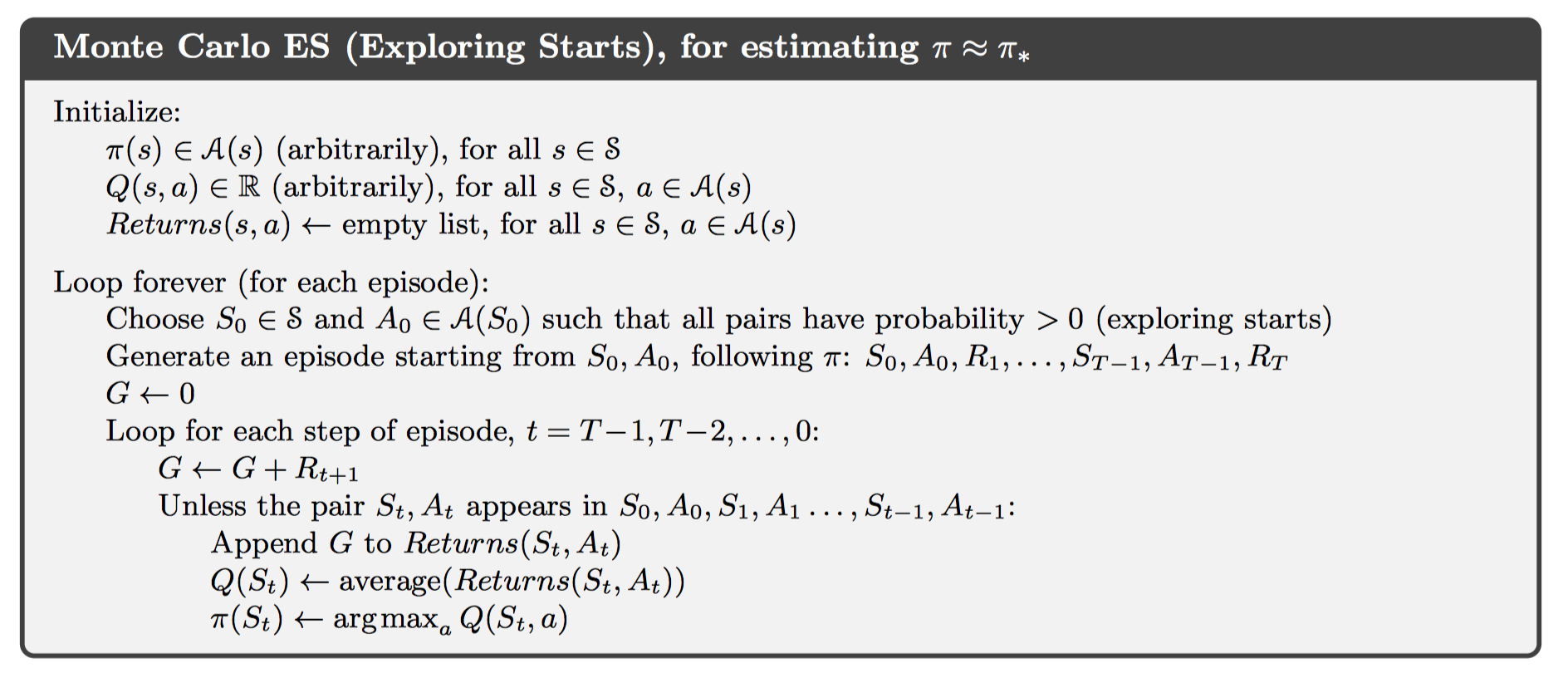On-policy via $\epsilon$-soft

MC方法中有两类方法可以良好地解决第一点问题，分别是on-policy, off-policy。这里首先介绍前者。

On-policy方法，一般来说都具有soft的特性，也就是$\pi(a|s)>0$。但是在策略优化步骤中将会逐渐得演变为确定性策略。下面给出算法实现：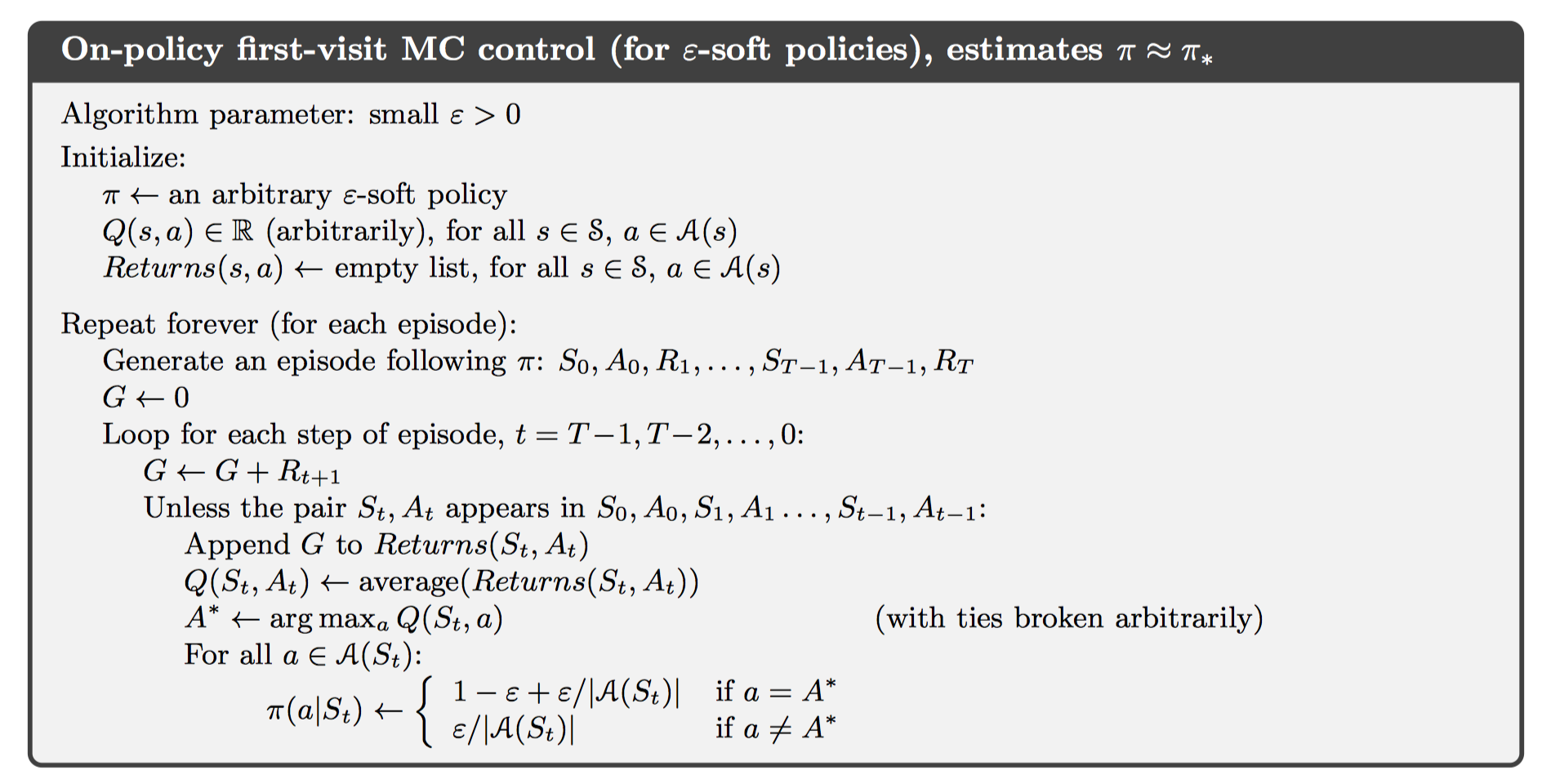\begin{equation} \begin{aligned} q_pi(s, \pi’(s)) &= \sum_a \pi’(a|s)q_\pi(s,a) \ &=\frac{\epsilon}{|A(s)|}\sum_aq_\pi(s,a) + (1-\epsilon)\max_aq_\pi(s,a) \ &\ge \frac{\epsilon}{|A(s)|}\sum_aq_\pi(s,a)+(1-\epsilon)\sum_a\frac{\pi(a|s) - \frac{\epsilon}{|A(s)|}}{1-\epsilon}q_\pi(s,a) \ &= \frac{\epsilon}{|A(s)|}\sum_aq_\pi(s,a)-\frac{\epsilon}{|A(s)|}\sum_aq_\pi(s,a) + \sum_a\pi(a|s)q_\pi(s,a) \ &= v_\pi(s) \end{aligned} \end{equation}

Oﬀ-policy Prediction via Importance Sampling

\begin{equation} \begin{aligned} \text{Pr}{&A_t, S_{t+1},,A_{t+1},\dots,S_T|S_t, A_{t:T-1~\sim \pi} }\ &=\pi(A_t|S_t)p(S_{t+1}|S_t, A_t)\pi(A_{t+1}|S_{t+1})\cdots p(S_T|S_{T-1},A_{T-1}) \ &= \prod_{k=t}^{T-1}\pi(A_k|S_k)p(S_{k+1}|S_k,A_k) \end{aligned} \end{equation}

$$\rho_{t:T-1} = \frac{\prod_{k=t}^{T-1}\pi(A_k|S_k)p(S_{k+1}|S_k,A_k)}{\prod_{k=t}^{T-1}b(A_k|S_k)p(S_{k+1}|S_k,A_k)} = \prod_{k=t}^{T-1}\frac{\pi(A_k|S_k)}{b(A_k|S_k)}$$

$$\mathbb{E}[\rho_{t:T-1}G_t|S_t] = v_\pi(S_t)$$

• ordinary importance sampling

这种方式对目标策略的估计公式为$V(s) = \displaystyle \frac{\sum_{t\in\mathcal{J}(s)}\rho_{t:T(t)-1}G_t}{|\mathcal{J}(s)|}$

表示对于每一次采样结果都平等对待。考虑当只有一次采样结果并且与实际值偏差较大的情况，那么可能会出现偏差非常大的结果。因此这是high variance的算法。

• weighted importance sampling

这种方式对目标策略的估计公式为$V(s) = \displaystyle \frac{\sum_{t\in\mathcal{J}(s)}\rho_{t:T(t)-1}G_t}{\sum_{t\in\mathcal{J}(s)}\rho_{t:T(t)-1}}$

如此一来，每一个采样结果将会根据importance-sampling ratio的值进行区别对待。因此不会再出现high variance的情况。

Oﬀ-policy MC prediction (policy evaluation)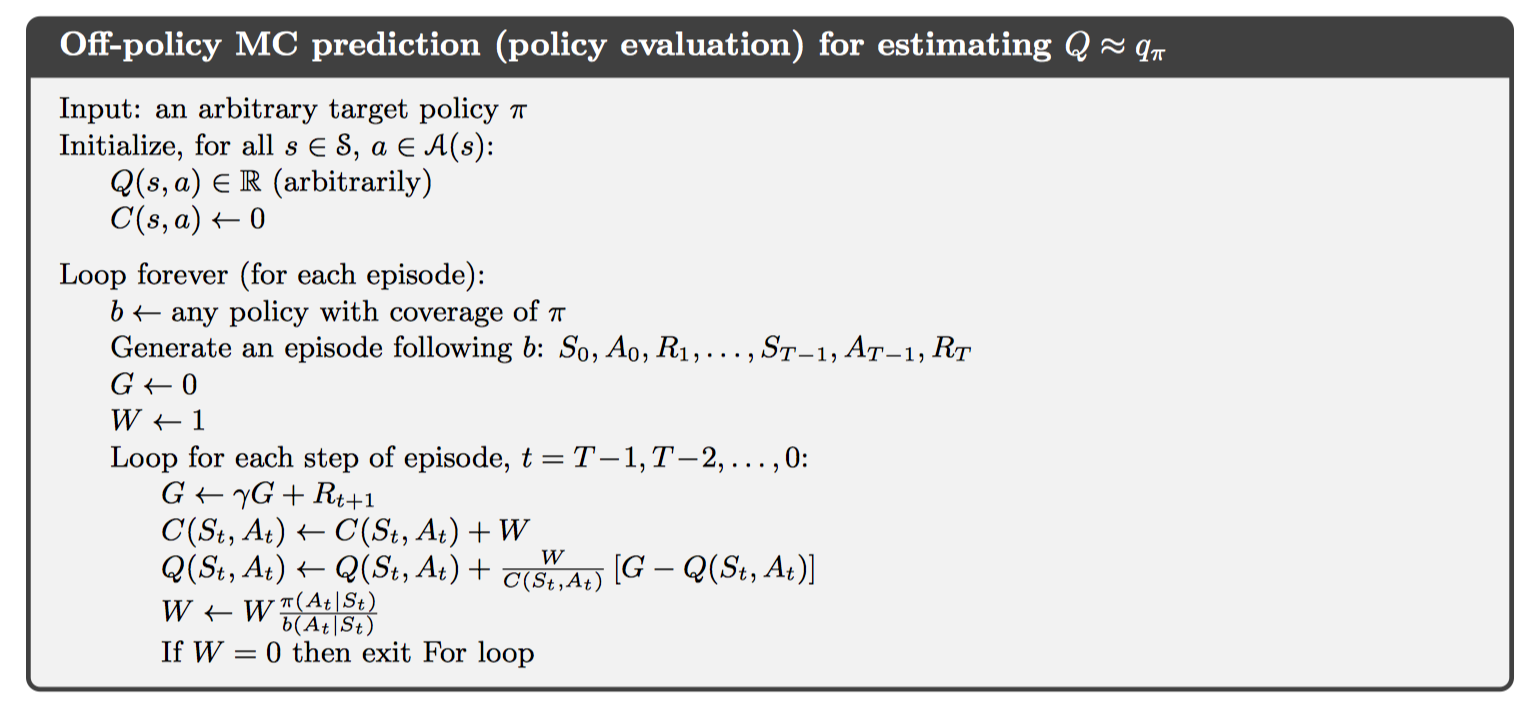Oﬀ-policy Monte Carlo Control(value iteration)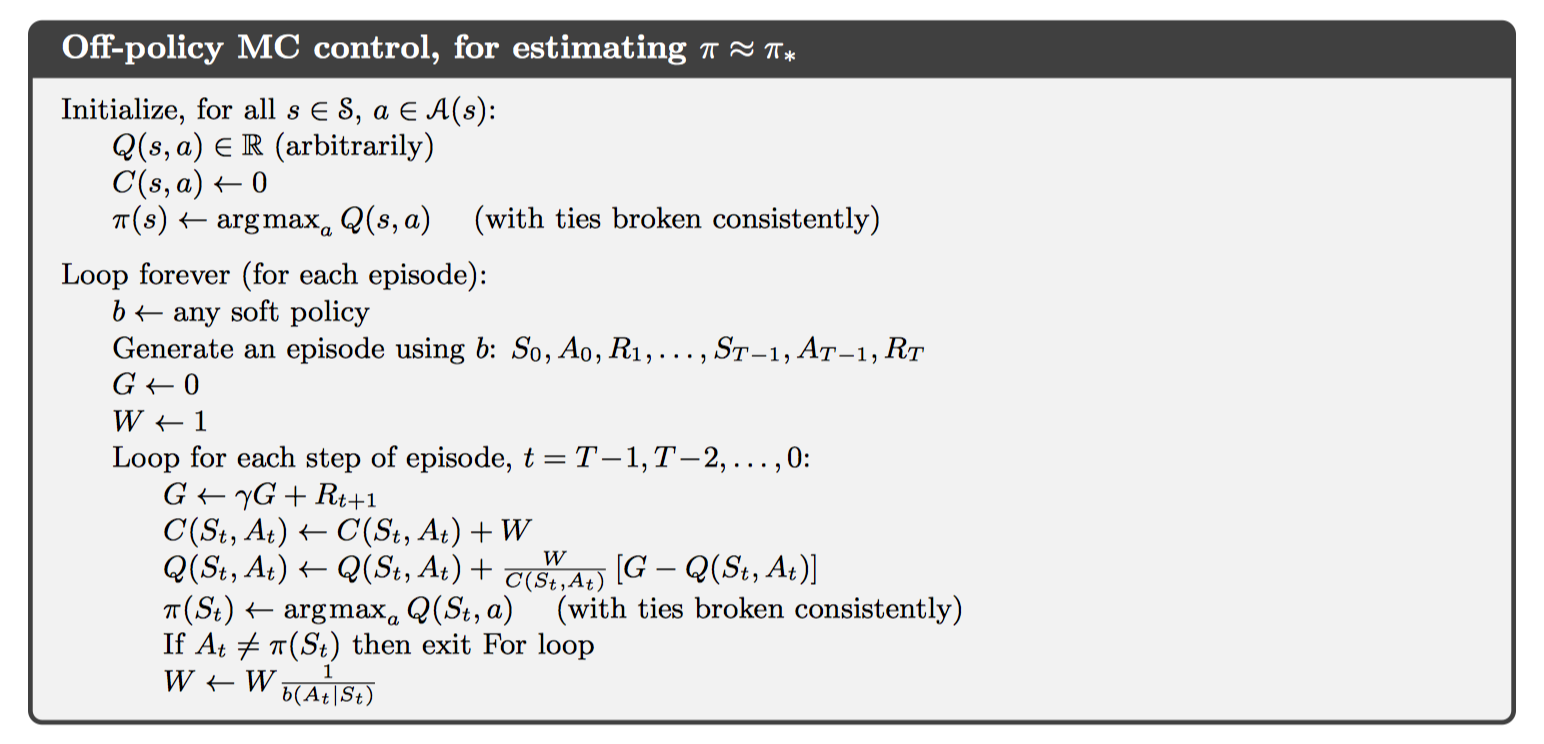0%